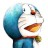# 杰拉斯的博客

## [转载]你真的已经搞懂JavaScript了吗？杰拉斯 | 时间：2012-12-02, Sun | 17,611 views

```if (!("a" in window)) {
var a = 1;
}

```var a = 1,
b = function a(x) {
x && a(--x);
};

```function a(x) {
return x * 2;
}
var a;

```function b(x, y, a) {
arguments = 10;
}
b(1, 2, 3);```

```function a() {
}
a.call(null);```

### 题目1

```if (!("a" in window)) {
var a = 1;
}

`"变量名称" in window`

```alert("a" in window);
var a;```

```var a;

```var a;    //声明
a = 1;    //初始化赋值```

```var a;
if (!("a" in window)) {
a = 1;
}

### 题目2

```var a = 1,
b = function a(x) {
x && a(--x);
};

```function functionName(arg1, arg2){
//函数体
}```

```var functionName = function(arg1, arg2){
//函数体
};```

```function value(){
return 1;
}
var value;

```function value(){
return 1;
}
var value = 1;

```var a = 1,
b = function(x) {
x && b(--x);
};

### 题目3

```function a() {
return 1 ;
}
var a;

### 题目4

```function b(x, y, a) {
arguments = 10;
}
b(1, 2, 3);```

### 题目5

```function a() {
}
a.call(null);```

```function a() {
}
a.call(window);```

—————

### 总结：

#### 仅有一条评论 »

1.Tater

拿去执行其实是最佳实践.